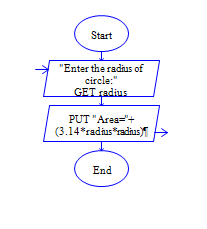# Area of Circle in Python

Python program to get radius of circle and find area of circle.

Sample Input 1:

4

Sample Output 1:

50.24

#### Flow Chart Design#### Program or Solution

``` r=int(input("Enter the radius:")) area=3.14*r*r print("area: {}".format(area)) ```

#### Program Explanation

get th radius of a circle using input() method calculate area = pi * r * r